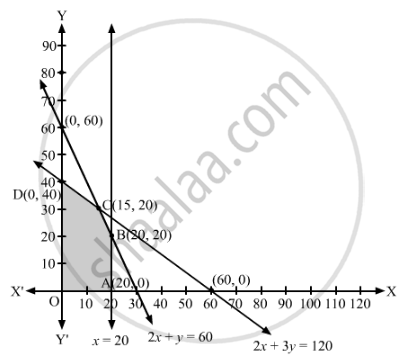# A Manufacturer Makes Two Types of Toys a and B. Three Machines Are Needed for this Purpose and the Time (In Minutes) Required for Each Toy on the Machines is Given Below: - Mathematics

Sum

A manufacturer makes two types of toys A and B. Three machines are needed for this purpose and the time (in minutes) required for each toy on the machines is given below:

 Types of Toys Machines I II III A 12 18 6 B 6 0 9

Each machine is available for a maximum of 6 hours per day. If the profit on each toy of type A is ₹7.50 and that on each toy of type B is ₹5, show that 15 toys of type A and 30 toys of type B should be manufactured in a day to get maximum profit.

#### Solution

Suppose the manufacturer makes x toys of type A and y toys of type B.

Since each toy of type A require 12 minutes on machine I and each toy of type B require 6 minutes on machine I, therefore, x toys of type A and y toys of type B require (12x + 6y) minutes on machine I. But, machines I is available for at most 6 hours.

∴ 12x + 6≤ 360

⇒ 2x + ≤ 60

Similarly, each toy of type A require 18 minutes on machine II and each toy of type B require 0 minutes on machine II, therefore, x toys of type A and y toys of type B require (18x + 0y) minutes on machine II. But, machines II is available for at most 6 hours.

∴ 18x + 0≤ 360

⇒ x ≤ 20

Also, each toy of type A require 6 minutes on machine III and each toy of type B require 9 minutes on machine III, therefore, x toys of type A and y toys of type B require (6x + 9y) minutes on machine III. But, machines III is available for at most 6 hours.

∴ 6x + 9≤ 360

⇒ 2x + 3≤ 120

The profit on each toy of type A is ₹7.50 and each toy of type B is ₹5. Therefore, the total profit from x toys of type A and y toys of type B is ₹(7.50+ 5y).

Thus, the given linear programming problem is

Maximise Z = 7.5+ 5y

subject to the constraints

2x + ≤ 60

x ≤ 20

2x + 3≤ 120

x, y ≥ 0

The feasible region determined by the given constraints can be diagrammatically represented as,The coordinates of the corner points of the feasible region are O(0, 0), A(20, 0), B(20, 20), C(15, 30) and D(0, 40).

The value of the objective function at these points are given in the following table.

 Corner Point Z = 7.5x + 5y (0, 0) 7.5 × 0 + 5 × 0 = 0 (20, 0) 7.5 × 20 + 5 × 0 = 150 (20, 20) 7.5 × 20 + 5 × 20 = 250 (15, 30) 7.5 × 15 + 5 × 30 = 262.5 → Maximum (0, 40) 7.5 × 0 + 5 × 40 = 200

The maximum value of Z is 262.5 at x = 15, y = 30.

Hence, 15 toys of type A and 30 toys of type B should be manufactured in a day to get maximum profit. The maximum profit is ₹262.50.

Concept: Graphical Method of Solving Linear Programming Problems
Is there an error in this question or solution?

#### APPEARS IN

RD Sharma Class 12 Maths
Chapter 30 Linear programming
Exercise 30.4 | Q 50 | Page 57